Wei, X., Sun, L., Zhou, H., Yang, Y., Wang, Y., and Gao, Y. (2020). "Propagation velocity model of stress waves in larch wood (Larix gmelinii) three-dimensional space with different moisture contents," BioRes. 15(3), 6680-6695.

#### Abstract

Based on the effects of stress wave propagation in larch (Larix gmelinii) wood, the propagation mechanism of stress wave was explored, and a theoretical model of the propagation velocity of stress waves in the three-dimensional space of wood was developed. The cross and longitudinal propagation velocities of stress wave were measured in larch wood under different moisture contents (46% to 87%, 56% to 96%, 20% to 62%, and 11% to 30%) in a laboratory setting. The relationships between the propagation velocity of stress waves and the direction angle or chord angle with different moisture contents were analyzed, and the three-dimensional regression models among four parameters were established. The analysis results indicated that under the same moisture content, stress wave velocity increased as the direction angle increased and decreased as chord angle increased, and the radial velocity was the largest. Under different moisture contents, stress wave velocity gradually decreased as moisture content increased, and the stress wave velocity was more noticeably affected by moisture content when moisture content was below the fiber saturation point (FSP, 30%). The nonlinear regression models of the direction angle, chord angle, moisture content, and the propagation velocity of stress wave fit the experiment data well (R2 ≥ 0.97).

Propagation Velocity Model of Stress Waves in Larch Wood (Larix gmelinii) Three-dimensional Space with Different Moisture Contents

Xiwen Wei,a,b Liping Sun,a,* Hongjv Zhou,a Yang Yang,c Yifan Wang,a and Yang Gao c

Based on the effects of stress wave propagation in larch (Larix gmelinii) wood, the propagation mechanism of stress wave was explored, and a theoretical model of the propagation velocity of stress waves in the three-dimensional space of wood was developed. The cross and longitudinal propagation velocities of stress wave were measured in larch wood under different moisture contents (46% to 87%, 56% to 96%, 20% to 62%, and 11% to 30%) in a laboratory setting. The relationships between the propagation velocity of stress waves and the direction angle or chord angle with different moisture contents were analyzed, and the three-dimensional regression models among four parameters were established. The analysis results indicated that under the same moisture content, stress wave velocity increased as the direction angle increased and decreased as chord angle increased, and the radial velocity was the largest. Under different moisture contents, stress wave velocity gradually decreased as moisture content increased, and the stress wave velocity was more noticeably affected by moisture content when moisture content was below the fiber saturation point (FSP, 30%). The nonlinear regression models of the direction angle, chord angle, moisture content, and the propagation velocity of stress wave fit the experiment data well (R≥ 0.97).

Keywords: Stress waves; Moisture content; Three-dimensional space; Propagation velocity model

Contact information: a: College of Mechanical and Electrical Engineering, Northeast Forestry University, Harbin, 150040, China; b: College of Electrical and Information Engineering, Heilongjiang University of Technology, Jixi, 158100, China; c: College of Art and Design, Harbin University, Harbin, 150040, China; *Corresponding author: zdhslp@163.com

INTRODUCTION

Stress waves are an important nondestructive tool for evaluating the quality and predicting the strength of wood. Stress wave detection is based on the relationships between sound propagation velocity (v), wood density (ρ), and elastic modulus (E) in wood (Randow and Gazonas 2009; Xu et al. 2018; Simic et al. 2019). Stress wave generation uses a pulse hammer to strike the transmitter sensor on the tested wood so that a mechanical stress wave is generated inside the wood and propagates under stress, and propagation time is measured by specific equipment to determine the elastic modulus of the wood (Feng-Lu et al. 2016; Liao et al. 2017).

Wood is hygroscopic, heterogeneous, and anisotropic. The propagation of stress waves in wood is affected by tree species, moisture content, and environmental temperature (Chauhan and Walker 2006; Chan et al. 2011; Liu et al. 2014; Yang et al. 2015). Xu et al. (2011) studied the effects of moisture content and temperature on the propagation velocity of stress waves in Korean pine wood. The work showed that moisture content is an important factor affecting stress wave propagation, and a regression model was established between the propagation velocity of stress wave and moisture content. Liu and Gao (2014) examined the longitudinal stress wave velocities of wood samples in different radial locations with different moisture content (0% to 65%). Yamasaki et al. (2017) investigated the effect of moisture content on the stress wave propagation velocity and obtained the relationship between the moisture content and the rate of change of velocity of full-size timber. This relationship was used to estimate the Young’s modulus of the timber in the air-drying state from the velocity in high-moisture condition. Yue et al. (2018) investigated on the effects of the seasonal temperature and moisture content on the electrical resistance of standing trees, and their combined effects on electrical resistance were analyzed, following which a regression model was also established. Cheng et al. (2020) developed a set of reformulated theoretical formulas to measure the relationships between moisture content and stress wave propagation velocity, dynamic modulus of elasticity (Ed), and modulus of stress-resistograph of the wood. The formulas can be used to quickly get the change trend of wood properties, and the calculated results were consistent with the experimental data.

In recent years, the theoretical model of stress wave propagation in wood has been thoroughly studied. Zhang et al. (2016) studied the propagation mechanism of stress waves in the cross-section and longitudinal section of Pinus resinosa and identified the time contour curve of stress wave propagation in standing trees. Li et al. (2014) examined stress wave velocity patterns in the cross-sections of black cherry trees, developed analytical models of stress wave velocity in healthy trees, and then tested the effectiveness of the models as a tool for tree decay diagnosis. Weng et al. (2016) established the propagation velocity model of stress wave in the radial section of wood and designed a four-way cross detection method to detect the internal defects of trees.

This study aimed to investigate stress wave propagation velocity in three-dimensional space with different moisture contents. Using the stress wave propagation velocity patterns in the cross and longitudinal sections of healthy trees as the foundation of the physical and mechanical properties of wood, a theoretical model of stress wave propagation velocity was obtained for different space angles, and the effect of moisture content on stress wave velocity of larch wood in different direction angles and chord angles was explored. In addition, regression models of stress wave propagation velocity, direction angle, chord angle, and moisture content in larch wood were established.

Theoretical Analysis of Stress Wave Propagation Velocity Model in Wood

Fibre direction influences many of the physical properties of wood. Among others, the electrical and thermal conductivity, swelling and shrinking, and the strength and stiffness properties strongly depend on the considered orientation relative to the fibre direction (Ehrhart et al. 2017; Liu et al. 2019). Wood is anisotropic, and in wood segments free of knots, bark inclusions, or any other local defect, wood is an approximately cylindrical material using the three main directions (longitudinal (L), tangential (T), and radial (R)) (Fig. 1).

Stress waves are assumed to propagate in the SE direction in the LR plane as shown in Fig. 1(b), in which S represents the signal transmitter and E represents the signal receiver. Given the angle (β) between SR and SEi.e., the direction angle, it is positive downward and negative upward. According to Hankinson’s wood diagonal compressive strength formula, the relationship between stress wave propagation velocity (V) and direction angle (β) was calculated with Eq. 1 (Mascia et al. 2011),

V(β) = vlv/ (v1cos2β + vrsin2β) (1)

where V(β) is the propagation velocity (m/s) of stress wave in LR plane, vl is longitudinal velocity (m/s), and vr is radial velocity (m/s). Equation 1 was approximated with the second-order Taylor polynomial, and the remainder was removed to obtain Eq. 2:

V(β) ≈ vvr(1 – vr/vl)β(2)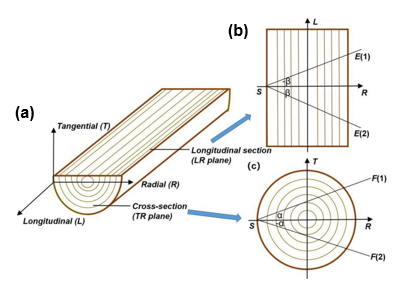Fig. 1. The cylindrical anisotropy of wood and the wood main directions longitudinal (L), tangential (T), and radial (R) on a wood plane (a); stress wave propagation in the LR plane (b) and the TR plane (c)

As shown in Fig. 1c, represents the signal transmitter, F represents the signal receiver, the angle between SR and SF is expressed by α, clockwise is negative, and counterclockwise is positive. The TR plane is considered a regular circle, and the stress wave propagates in the direction of SF. The propagation velocity in the SR direction is the radial velocity (α = 0), and the propagation velocity in the other directions is called chord velocity. According to Dikrallah et al. (2006), the acoustic anisotropy of wet wood was analyzed via a guided wave experiment, and the propagation of stress waves in the cross-section of wood was studied. The mathematical relationship between stress wave propagation velocity (V) and the chord angle α was obtained with Eq. 3,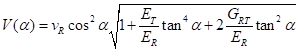(3)

where V(α) is the propagation velocity of stress wave in TR plane, vis radial velocity (α = 0), and ETER, and GRT represent the radial modulus of elasticity, tangential modulus of elasticity, and shear modulus, respectively.

According to Eq. 3, V(0) = vRV’(0) = 0, V’’(0) = -2vR(1 – GRTER), and the function V(α) could be expanded to Taylor series at α = 0 with Eq. 4:

V(α) = vR[1-(1 – GRTER)α2] (4)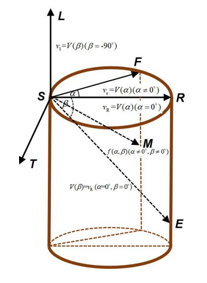Fig. 2. The propagation diagram of stress wave in three-dimensional coordinate system of wood

In cylindrical wood, a three-dimensional coordinate system was established with S as the origin, as shown in Fig. 2. The propagation velocities of stress wave, such as vrvlvRV(β) and V(α) are represented in vector form in three-dimensional coordinate system. The V(α) of Eq. 4 is the vr of Eq. 2, and Eq. 4 is substituted into Eq. 2. Let f(α, β) represent the simplified formula; the propagation velocity model of stress waves in three-dimensional space was obtained with Eq. 5: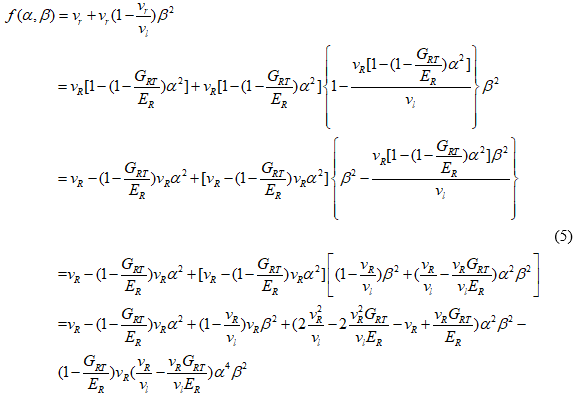When wood is approximately cylindrical, the propagation velocity f(α, β) is the sum of polynomials with the highest degree of 4 in direction angle (β) and chord angle (α), and it is symmetric about α = 0, β = 0. To simplify the theoretical model, the high-order terms in the formula were ignored, and the simplified formula (Eq. 6) was used:

f(α,β) ≈ vR +(1 – vRvl)vRβ2-(1 – GRTER)vRα(6)

In this paper, Eq. 6 was used as the basic model for the propagation velocity of stress waves in the three-dimensional space of healthy wood. The α and β values were used to determine the specific direction of stress wave propagation in wood space, and the GRT and ER values were determined by the mechanical properties of wood itself, which were obtained according to different wood species. The vR and vl were obtained by experimental measurement.

However, moisture content notably affects the propagation velocity of stress waves in wood, and the stress wave propagation velocity decreases gradually as moisture content increases (Gao et al. 2017). When the moisture content was introduced into the basic model (Eq. 6) as a variable, a more complete model of the propagation velocity of the stress wave in the three-dimensional space was established to obtain a more accurate space propagation velocity of the larch wood.

EXPERIMENTAL

Materials

Larch (Larix gmelinii) was obtained from the experimental forest farm of Northeast Forestry University (Harbin, China). Samples were selected that were free of nodes, decay, and cracking, they were felled, and branches were subsequently removed. Four 0.7-m-long wood pieces were cut from four sample trees of uniform thickness at a height of 0.6 m above the ground, and the diameter at breast height (DBH) values of the specimens were 20 cm, 22 cm, 22 cm, and 23 cm. The four 0.7-m-long wood specimens were numbered (#1, #2, #3, and #4) and immediately sealed in plastic wrap. Next, the wood specimens were directly transported to the mechanics laboratory of Northeast Forestry University and kept in a conditioned room at 20 °C.

Methods

Stress wave velocity measurement

Twelve test positions were selected on both sides of the longitudinal sections on each of the larch specimens at intervals of 10 cm. Pins were fixed onto each test position, and the pins were numbered No. 1 to No. 12. The nondestructive stress wave tester (Arbotom, Frank Rinn, Germany) was used to measure the stress wave velocity. The sensors were attached at the pins of each sample. After striking the sensors on one side, the transmitted stress wave was detected by the sensors on the other side of each specimen, and the direction angle between the contralateral sensors was represented by β. Then, sensors No. 1 to No. 6 were fixed, and sensors No. 7 to No. 12 were changed so that the angle between the longitudinal section and the radial section was expressed by α. The values of α were 0, ± 15°, ± 30°, ± 45°, ± 60°, and ±75°, and the stress wave velocities in 11 different longitudinal sections were measured; the same longitudinal section was measured 5 times and averaged. The equipment for stress wave testing is shown in Fig. 3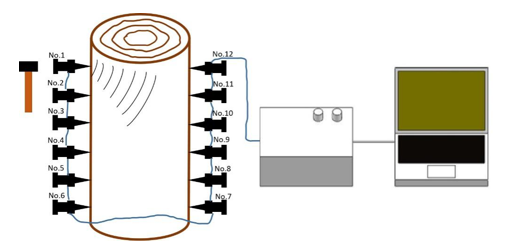Fig. 3. Schematic diagram of nondestructive stress wave testing of wood

Moisture content measurement

The volume of wood specimens is relatively large. A high frequency wood moisture meter (FD-100B; Shanghai Yuanqi Testing Instrument Co., Ltd., Shanghai, China) was used to quickly determine the moisture content of samples, and the measurement range was 0 to 100%. The initial moisture contents of the samples (#1, #2, #3, and #4) were estimated using the direct measurements taken by the moisture meter, and then samples #1 and #2 were immersed in water to improve their moisture contents. Samples were then taken at 5 intervals to measure the moisture content and the stress wave propagation velocity. Samples #3 and #4 were dried in a self-made experimental drying kiln of Northeast Forestry University, which could detect the moisture content of the wood samples in real time. When the moisture content reached the preset value, the samples were taken out to verify the moisture content with an FD-100B and measure the propagation velocity of the stress wave. The samples were also taken out 5 times and recorded during this part of the experiment. The measured moisture contents of the samples (#1, #2, #3, and #4) are shown in Table 1.

Table 1. Sample Moisture Content Measurement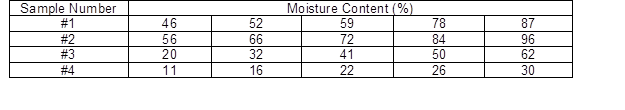Statistical analysis method

Based on the measured data, drawing software (Origin, OriginLab, v.9.0, Northampton, MA, USA) and statistical analysis software (SPSS, IBM, IBM SPSS Statistics 26.0, Armonk, NY, USA) were used to analyze the propagation velocity of stress wave in the longitudinal section and the cross-section with different moisture contents, and establish the corresponding regression model. Matlab software (MATLAB, MathWorks, MATLAB R2017b, Natick, MA, USA) was used to analyze the comprehensive influence law of stress wave propagation velocity in different moisture contents and space angles and draw three-dimensional curved surface.

RESULTS AND DISCUSSION

Analysis of the Longitudinal Section Velocity of Stress Wave with Different Moisture Contents

When the chord angle (α) of larch was 0, the propagation velocity of the stress waves at different direction angles (β) between sensor No. 3 and sensors No.7 to No.12 were measured on the wood samples (#1, #2, #3, and #4) with different moisture contents. The results are shown in Fig. 4.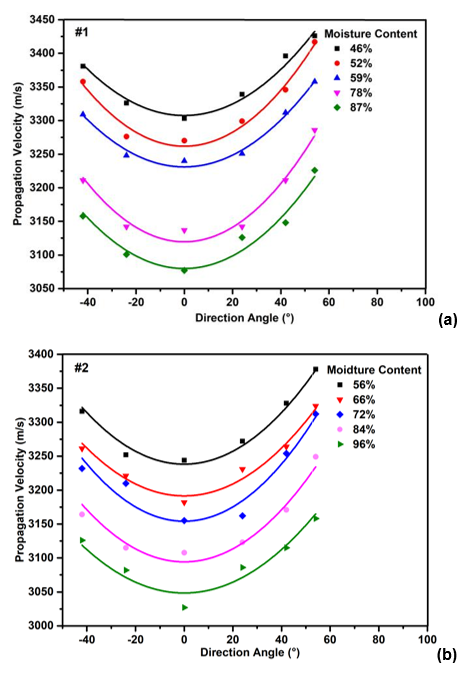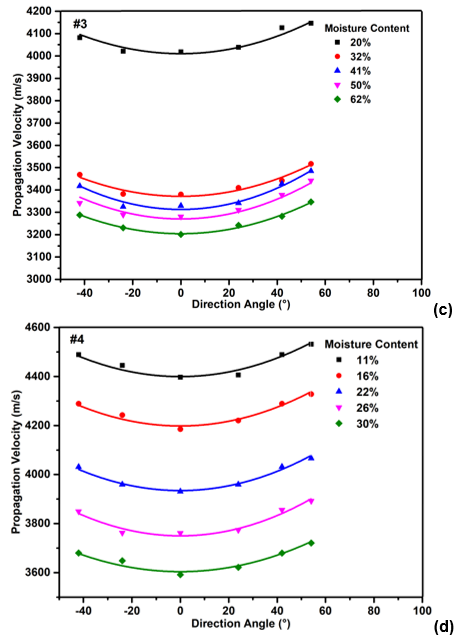Fig. 4. Effect of direction angle on stress wave velocity with (a) 46% to 87% moisture contents, (b) 56% to 96% moisture contents, (c) 20% to 62% moisture contents, and (d) 11% to 30% moisture contents

Figure 4 shows that when the moisture content of larch wood samples was different, the effects of directional angle change on the propagation velocity of stress wave were similar. When the propagation velocity increased with increasing direction angle, the horizontal or radial (α = 0) velocity was the smallest, and the overall trend was an upward parabola from the opening that was symmetrical about 0. The propagation velocity of the stress wave gradually decreased as moisture content increased, and at the moisture content of approximately 30%, the propagation velocity changed greatly (Fig. 4), and the stress wave velocity was more noticeably affected by moisture content when moisture content was below 30%.

Table 2. Regression Analysis between Stress Wave Velocity and Direction Angle with Different Larch Moisture Contents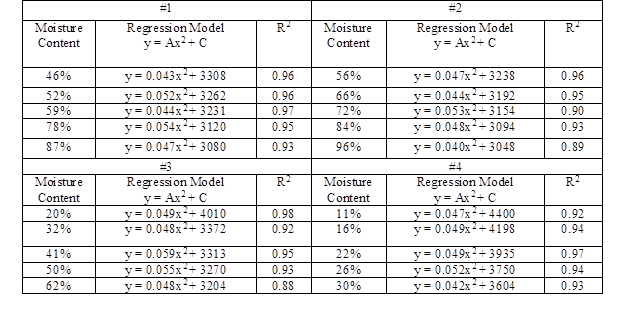The fiber saturation point (FSP) is an important concept in wood-moisture relations. The FSP can be defined as the moisture content of wood when only the bound water in cell wall reaches saturation, but there is no free water in cell cavity and intercellular space. It was an important boundary point for the changes in wood physical and mechanical properties (Stamm 1929; Skaar 1988). Below the FSP, there was no free water in the cell cavity of wood, whereas almost all the changes of physical and mechanical properties of wood mainly occur in the change stage of bound water content in the cell wall, and the drying shrinkage and swelling of wood basically depend on the change of bound water content. Above the FSP, the bound water and free water coexist. Thus, the composition of wood includes the free water and wood body with the fiber saturation point. The change of water content was the increase or decrease of free water in the cell cavity, which has no effect on the physical properties of wood except for its mass (Gao and Wang 2008). At present, the FSP commonly used in most textbooks is 23% to 33%, with an average of 30% (Gao et al. 2019). The propagation velocity of stress wave in wood is affected by the physical and mechanical properties of wood. Therefore, FSP is also an important boundary point that affects the change of stress wave velocity.

SPSS software was used to conduct regression analysis on the data of stress wave velocity and direction angle according to the mathematical model (y = Ax2 + C) of Eq. 2, and regression models between them were established (Table 2). For all moisture contents of the larch wood samples, the regression curve of stress wave velocity and direction angle satisfied the one-dimensional quadratic function relation, and the determination coefficients (R2) were above 0.88, which indicated that the models had high relation.

Analysis of the Cross-section Velocity of Stress Wave with Different Moisture Contents

When the direction angle β of larch was 0, the propagation velocity of the stress waves of different chord angles α were measured on the wood samples (#1, #2, #3, and #4) with different moisture content. The results are shown in Fig. 5.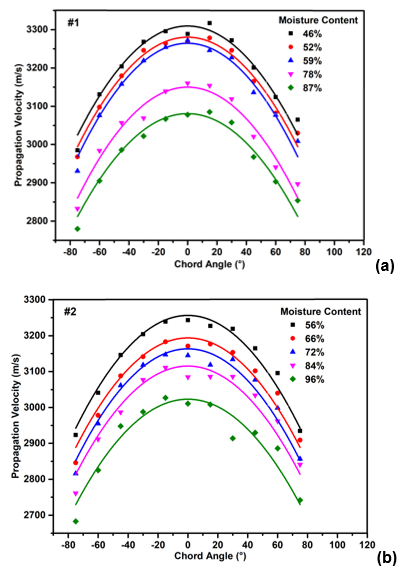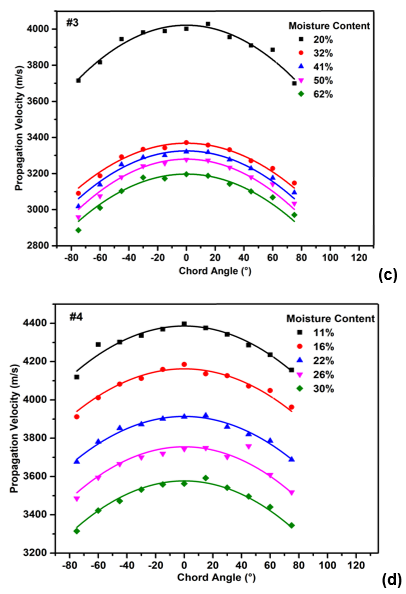Fig. 5. Effect of chord angle on stress wave velocity with (a) 46% to 87% moisture contents, (b) 56% to 96% moisture contents, (c) 20% to 62% moisture contents, and (d) 11% to 30% moisture contents

Table 3. Regression Analysis between Stress Wave Velocity and Chord Angle with Different Larch Moisture Contents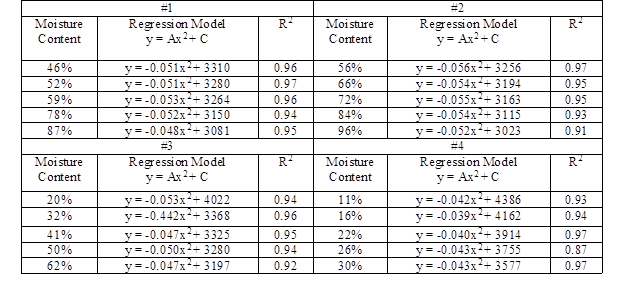Figure 5 shows that the effects of the variation of chord angle on the stress wave velocity of larch wood samples with different moisture content were similar. The stress wave propagation velocity decreased as chord angle increased, and the radial propagation velocity was the largest. The overall trend was a downward parabola from the opening that was symmetrical about 0. Similarly, the propagation velocity decreased as the moisture content in the cross-sections increased, and the variation range of moisture content above the FSP was smaller than that below the FSP. For the regression analysis shown in Table 3, in accordance with the mathematical model (y = Ax2 + C) of Eq. 4, the determination coefficients (R2) were above 0.87, which indicated that the models had high relation.

A stress wave, as an elastic mechanical wave, has different propagation velocity in different directions of the same tree species when it propagates in wood. When the tree rings were uniform, the material is dense and the texture is straight, the cut material is uniform, and the tubular cells are arranged neatly and straightly, which is beneficial to the propagation of elastic waves, that is, the stress wave propagates fastest in the direction of the same wood, and the radial propagation velocity under the transverse stripes is greater than the chord propagation velocity.

Analysis of the Three-dimensional Velocity of Stress Wave with Different Moisture Contents

The comprehensive influence of stress wave propagation velocity of the samples #1 and #4 with different moisture contents and space angles are shown in Fig. 6. The three-dimensional surface maps showed that the interaction between the direction angle, chord angle, and moisture content had a great influence on the stress wave velocity, and the propagation velocity of the stress wave propagated symmetrically with the direction angle and the chord angle. Under different moisture contents, the regression models of stress wave propagation velocity and space angles were established according to the mathematical model (z = Ax2 + By2 + C) of Eq. 6. As the R2 coefficients were above 0.97 and the significance (P) was less than 0.01, the models had high relation. Analysis of the models showed that variation in moisture content had small effects on coefficients A and B and had a large effect on C.